# In a circuit consisting of a capacitance and a generator with alternating emfQuestion:

In a circuit consisting of a capacitance and a generator with alternating emf $E_{g}=E_{g_{0}} \sin \omega t, V_{C}$ and $\mathrm{I}_{\mathrm{C}}$ are the voltage and current. Correct phasor diagram for such circuit is :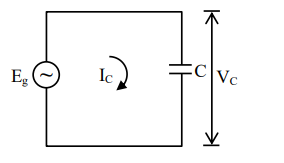1.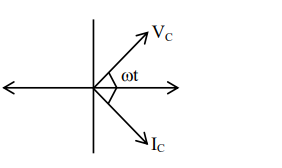2.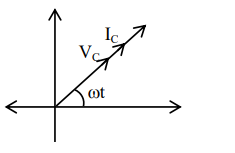3.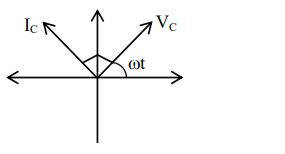4.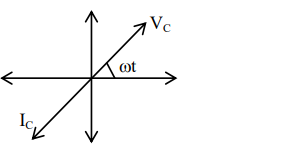Correct Option: , 3

Solution:

In capacitor, current lead voltage by $\frac{\pi}{2}$